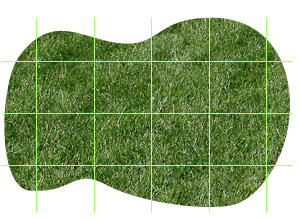# Lawn Area Calculator

### Square or rectangular lawns

To measure a square or rectangular lawn, multiply the length by the width.Area: 3m x 2m = 6m2

### L-shaped Lawn

If your lawn is L-shaped or similar, divide it into rectangles and add the areas of each rectangle using the Area Calculator.Area A: 1.5m x 1m = 1.5m2

Area B: 3m x 1m = 3m2

Total area: A + B = 4.5m2

### Odd-shaped lawns

If your lawn is an odd shape, an easy way to measure it is to draw it on graph paper. Take measurements at key points of the lawn (such as the widest and longest points), plot the points on graph paper, with each graph square representing 1 metre, and sketch the approximate outline of the lawn. Count the number of full squares, estimate the area of the partially filled squares, and add the amounts for an approximate total area. If in doubt, it is better to overestimate the area than to underestimate it.8 full squares = 8m2

16 partially-filled squares = approx. 9m2

Total area = 17m2